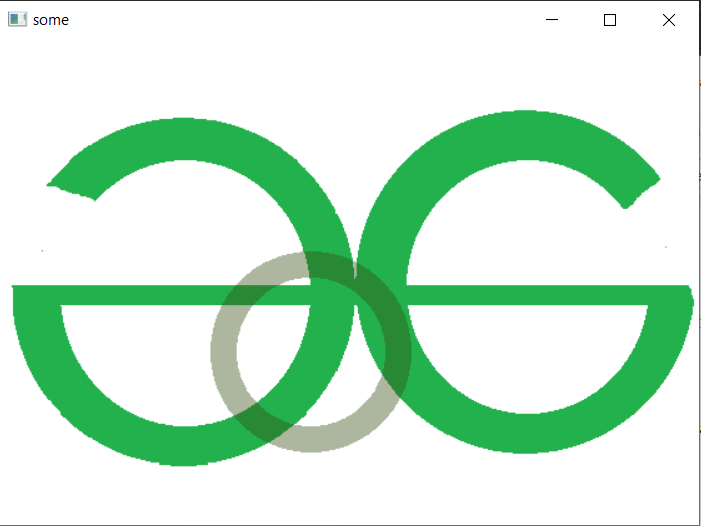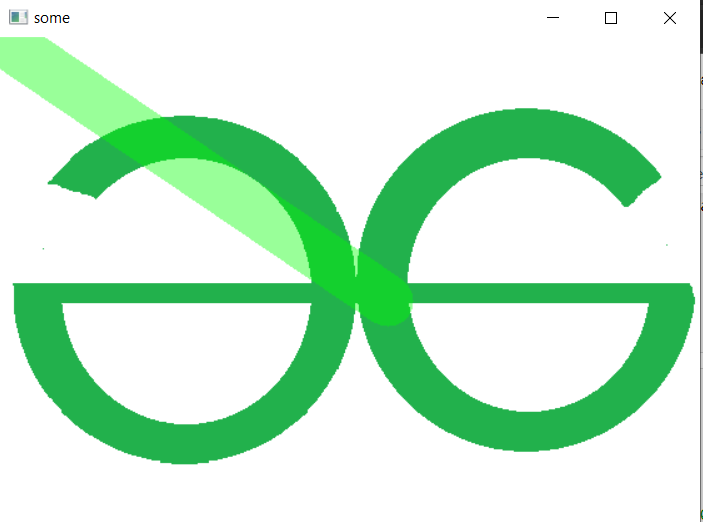# How to create a semi transparent shape Python-OpenCV

• Last Updated : 22 Apr, 2022

In this article, we will see how to create a semi-transparent shape python OpenCV.

Sometimes we need transparency in our outputs. It gives our outputs their own unique style. Today, we will see how you can do it easily with OpenCV python. Here, we are going to cover 3 different methods of shape and they are:

• Using Rectangular shape.
• Using Line.
• and, Using Circle.

Note: The concept will be the same for any other shape or image.

## Method 1: Using cv2.rectangle

To draw a rectangle in Opencv Python.

Syntax: cv2.rectangle(image, start_point, end_point, color, thickness)

Parameters:

• image: It is the image on which line is to be drawn.
• start_point: It is the starting coordinates of line. The coordinates are represented as tuples of two values i.e. (X coordinate value, Y coordinate value).
• end_point: It is the ending coordinates of line. The coordinates are represented as tuples of two values i.e. (X coordinate value, Y coordinate value).
• color: It is the color of line to be drawn. For BGR, we pass a tuple. eg: (255, 0, 0) for blue color.
• thickness: It is the thickness of the line in px.

Return Value: It returns an image.

Example 1: Without opaque

## Python3

 `import` `cv2 ` ` `  `image ``=` `cv2.imread(``'test.jpg'``) ` `overlay ``=` `image.copy() ` ` `  `# Rectangle parameters ` `x, y, w, h ``=` `10``, ``10``, ``300``, ``300` ` `  `# A filled rectangle ` `cv2.rectangle(overlay, (x, y), (x``+``w, y``+``h), (``0``, ``200``, ``0``), ``-``1``) ` ` `  `cv2.imshow(``"some"``, overlay) ` `cv2.waitKey(``0``) ` ` `  `cv2.destroyAllWindows()`

Output:Example 2: With opaque

For adding opacity we are going to use cv2.addWeighted. This helps us in adding 2 images with different weights of alpha.

## Python3

 `import` `cv2 ` ` `  `image ``=` `cv2.imread(``'test.jpg'``) ` `overlay ``=` `image.copy() ` ` `  `# Rectangle parameters ` `x, y, w, h ``=` `10``, ``10``, ``300``, ``300`   `# A filled rectangle ` `cv2.rectangle(overlay, (x, y), (x``+``w, y``+``h), (``0``, ``200``, ``0``), ``-``1``)   ` ` `  `alpha ``=` `0.4`  `# Transparency factor. ` ` `  `# Following line overlays transparent rectangle ` `# over the image ` `image_new ``=` `cv2.addWeighted(overlay, alpha, image, ``1` `-` `alpha, ``0``) ` ` `  `cv2.imshow(``"some"``, image_new) ` `cv2.waitKey(``0``) ` ` `  `cv2.destroyAllWindows() `

Output:## Method 2: Using cv2.circle

To draw a circle in Opencv Python

Syntax: cv2.circle(image, center_coordinates, radius, color, thickness)

Parameters:

• image: It is the image on which line is to be drawn.
• center_coordinates: It is the center coordinates of circle. The coordinates are represented as tuples of two values i.e. (X coordinate value, Y coordinate value).
• color: It is the color of line to be drawn. For BGR, we pass a tuple. eg: (255, 0, 0) for blue color.
• thickness: It is the thickness of the line in px.

Return Value: It returns an image.

Example: Here, we will draw a circle in Opencv Python. In this, we will pass center_coordinates and radius where the center coordinate is represented as tuples of two values of X-coordinate and Y-coordinate values.

## Python3

 `import` `cv2 ` ` `  `image ``=` `cv2.imread(``'geekslogo.png'``) ` `overlay ``=` `image.copy() ` ` `  `# A filled circle ` `cv2.circle(overlay, (``250``, ``250``), ``70``, (``15``,``75``,``50``), ``20``)    ` ` `  `alpha ``=` `0.4`  `# Transparency factor. ` ` `  `# Following line overlays transparent rectangle ` `# over the image ` `image_new ``=` `cv2.addWeighted(overlay, alpha, image, ``1` `-` `alpha, ``0``) ` ` `  `cv2.imshow(``"some"``, image_new) ` `cv2.waitKey(``0``) ` ` `  `cv2.destroyAllWindows() `

Output:## Method 3:  Using cv2.line

To draw a line in Opencv Python,

Syntax: cv2.line(image, start_point, end_point, color, thickness)

Parameters:

• image: It is the image on which line is to be drawn.
• start_point: It is the starting coordinates of line. The coordinates are represented as tuples of two values i.e. (X coordinate value, Y coordinate value).
• end_point: It is the ending coordinates of line. The coordinates are represented as tuples of two values i.e. (X coordinate value, Y coordinate value).
• color: It is the color of line to be drawn. For BGR, we pass a tuple. eg: (255, 0, 0) for blue color.
• thickness: It is the thickness of the line in px.

Return Value: It returns an image.

Example: Here, we will draw a line in Opencv Python. In this, we will pass start_point and end_point where both are represented as tuples of two values of X-coordinate and Y-coordinate values.

## Python3

 `import` `cv2 ` ` `  `image ``=` `cv2.imread(``'geekslogo.png'``) ` `overlay ``=` `image.copy() ` ` `  `# A filled line ` `cv2.line(overlay, (``0``,``0``), (``311``,``211``), (``0``,``355``,``0``),``40``) ` ` `  `# Transparency factor. ` `alpha ``=` `0.4`   ` `  `# Following line overlays transparent rectangle  ` `# over the image ` `image_new ``=` `cv2.addWeighted(overlay, alpha, image, ``1` `-` `alpha, ``0``) ` ` `  `cv2.imshow(``"some"``, image_new) ` `cv2.waitKey(``0``) ` ` `  `cv2.destroyAllWindows() `

Output:My Personal Notes arrow_drop_up
Recommended Articles
Page :